CAT  >  Test: Cubes & Cuboids

# Test: Cubes & Cuboids

Test Description

## 31 Questions MCQ Test Logical Reasoning (LR) and Data Interpretation (DI) | Test: Cubes & Cuboids

Test: Cubes & Cuboids for CAT 2023 is part of Logical Reasoning (LR) and Data Interpretation (DI) preparation. The Test: Cubes & Cuboids questions and answers have been prepared according to the CAT exam syllabus.The Test: Cubes & Cuboids MCQs are made for CAT 2023 Exam. Find important definitions, questions, notes, meanings, examples, exercises, MCQs and online tests for Test: Cubes & Cuboids below.
Solutions of Test: Cubes & Cuboids questions in English are available as part of our Logical Reasoning (LR) and Data Interpretation (DI) for CAT & Test: Cubes & Cuboids solutions in Hindi for Logical Reasoning (LR) and Data Interpretation (DI) course. Download more important topics, notes, lectures and mock test series for CAT Exam by signing up for free. Attempt Test: Cubes & Cuboids | 31 questions in 30 minutes | Mock test for CAT preparation | Free important questions MCQ to study Logical Reasoning (LR) and Data Interpretation (DI) for CAT Exam | Download free PDF with solutions
 1 Crore+ students have signed up on EduRev. Have you?
Test: Cubes & Cuboids - Question 1

### Directions to Solve The following questions are based on the information given below: A cuboid shaped wooden block has 6 cm length, 4 cm breadth and 1 cm height. Two faces measuring 4 cm x 1 cm are coloured in black. Two faces measuring 6 cm x 1 cm are coloured in red. Two faces measuring 6 cm x 4 cm are coloured in green. The block is divided into 6 equal cubes of side 1 cm (from 6 cm side), 4 equal cubes of side 1 cm(from 4 cm side). Question - How many cubes having red, green and black colours on at least one side of the cube will be formed ?

Detailed Solution for Test: Cubes & Cuboids - Question 1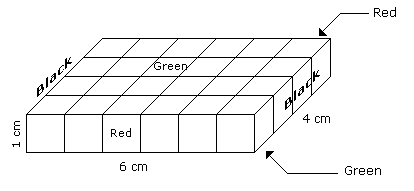Such cubes are related to the corners of the cuboid. Since the number of corners of the cuboid is 4.

Hence, the number of such small cubes is 4.

Test: Cubes & Cuboids - Question 2

### Directions to Solve The following questions are based on the information given below: All the opposite faces of a big cube are coloured with red, black and green colours. After that is cut into 64 small equal cubes. Question - How many small cubes are there where one face is green and other one is either black or red ?

Detailed Solution for Test: Cubes & Cuboids - Question 2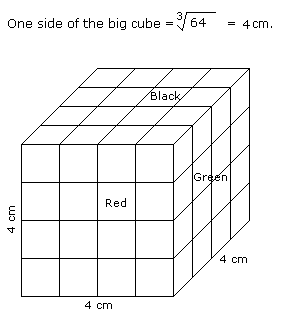Number of small cubes having one face green and the other one is either red or black = 8 x 2 = 16

Test: Cubes & Cuboids - Question 3

### Directions to Solve The following questions are based on the information given below: A cuboid shaped wooden block has 6 cm length, 4 cm breadth and 1 cm height. Two faces measuring 4 cm x 1 cm are coloured in black. Two faces measuring 6 cm x 1 cm are coloured in red. Two faces measuring 6 cm x 4 cm are coloured in green. The block is divided into 6 equal cubes of side 1 cm (from 6 cm side), 4 equal cubes of side 1 cm(from 4 cm side). Question - How many small cubes will be formed ?

Detailed Solution for Test: Cubes & Cuboids - Question 3Number of small cubes = l x b x h = 6 x 4 x 1 = 24

Test: Cubes & Cuboids - Question 4

Directions to Solve

The following questions are based on the information given below:

1. A cuboid shaped wooden block has 6 cm length, 4 cm breadth and 1 cm height.
2. Two faces measuring 4 cm x 1 cm are coloured in black.
3. Two faces measuring 6 cm x 1 cm are coloured in red.
4. Two faces measuring 6 cm x 4 cm are coloured in green.
5. The block is divided into 6 equal cubes of side 1 cm (from 6 cm side), 4 equal cubes of side 1 cm(from 4 cm side).

Question -

How many cubes will have 4 coloured sides and two non-coloured sides ?

Detailed Solution for Test: Cubes & Cuboids - Question 4Only 4 cubes situated at the corners of the cuboid will have 4 coloured and 2 non-coloured sides.

Test: Cubes & Cuboids - Question 5

Directions to Solve

The following questions are based on the information given below:

1. A cuboid shaped wooden block has 6 cm length, 4 cm breadth and 1 cm height.
2. Two faces measuring 4 cm x 1 cm are coloured in black.
3. Two faces measuring 6 cm x 1 cm are coloured in red.
4. Two faces measuring 6 cm x 4 cm are coloured in green.
5. The block is divided into 6 equal cubes of side 1 cm (from 6 cm side), 4 equal cubes of side 1 cm(from 4 cm side).

Question -

How many cubes will remain if the cubes having black and green coloured are removed ?

Detailed Solution for Test: Cubes & Cuboids - Question 5Number of small cubes which are Black and Green is 8 in all.

Hence, the number of remaining cubes are = 24 - 8 = 16

Test: Cubes & Cuboids - Question 6

Directions to Solve

The following questions are based on the information given below:

1. A cuboid shaped wooden block has 6 cm length, 4 cm breadth and 1 cm height.
2. Two faces measuring 4 cm x 1 cm are coloured in black.
3. Two faces measuring 6 cm x 1 cm are coloured in red.
4. Two faces measuring 6 cm x 4 cm are coloured in green.
5. The block is divided into 6 equal cubes of side 1 cm (from 6 cm side), 4 equal cubes of side 1 cm(from 4 cm side).

Question -

How many cubes will have green colour on two sides and rest of the four sides having no colour ?

Detailed Solution for Test: Cubes & Cuboids - Question 6There are 16 small cubes attached to the outer walls of the cuboid.

Therefore remaining inner small cubes will be the cubes having two sides green coloured.

So the required number = 24 - 16 = 8

Test: Cubes & Cuboids - Question 7

Directions to Solve

The following questions are based on the information given below:

1. There is a cuboid whose dimensions are 4 x 3 x 3 cm.
2. The opposite faces of dimensions 4 x 3 are coloured yellow.
3. The opposite faces of other dimensions 4 x 3 are coloured red.
4. The opposite faces of dimensions 3 x 3 are coloured green.
5. Now the cuboid is cut into small cubes of side 1 cm.

Question -

How many small cubes will have only two faces coloured ?

Detailed Solution for Test: Cubes & Cuboids - Question 7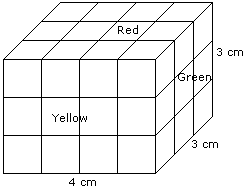Number of small cubes having only two faces coloured = 6 from the front + 6 from the back + 2 from the left + 2 from the right

= 16

Test: Cubes & Cuboids - Question 8

Directions to Solve

The following questions are based on the information given below:

1. There is a cuboid whose dimensions are 4 x 3 x 3 cm.
2. The opposite faces of dimensions 4 x 3 are coloured yellow.
3. The opposite faces of other dimensions 4 x 3 are coloured red.
4. The opposite faces of dimensions 3 x 3 are coloured green.
5. Now the cuboid is cut into small cubes of side 1 cm.

Question -

How many small cubes have three faces coloured ?

Detailed Solution for Test: Cubes & Cuboids - Question 8Such cubes are related to the corners of the cuboid and there are 8 corners.

Hence, the required number is 8.

Test: Cubes & Cuboids - Question 9

Directions to Solve

The following questions are based on the information given below:

1. There is a cuboid whose dimensions are 4 x 3 x 3 cm.
2. The opposite faces of dimensions 4 x 3 are coloured yellow.
3. The opposite faces of other dimensions 4 x 3 are coloured red.
4. The opposite faces of dimensions 3 x 3 are coloured green.
5. Now the cuboid is cut into small cubes of side 1 cm.

Question -

How many small cubes will have no face coloured ?

Detailed Solution for Test: Cubes & Cuboids - Question 9Number of small cubes have no face coloured = (4 - 2) x (3 - 2) = 2 x 1 = 2

Test: Cubes & Cuboids - Question 10

Directions to Solve

The following questions are based on the information given below:

1. There is a cuboid whose dimensions are 4 x 3 x 3 cm.
2. The opposite faces of dimensions 4 x 3 are coloured yellow.
3. The opposite faces of other dimensions 4 x 3 are coloured red.
4. The opposite faces of dimensions 3 x 3 are coloured green.
5. Now the cuboid is cut into small cubes of side 1 cm.

Question -

How many small cubes will have only one face coloured ?

Detailed Solution for Test: Cubes & Cuboids - Question 10Number of small cubes having only one face coloured = 2 x 2 + 2 x 2 + 2 x 1

= 4 + 4 + 2

= 10

Test: Cubes & Cuboids - Question 11

Directions to Solve

The following questions are based on the information given below:

1. A cuboid shaped wooden block has 4 cm length, 3 cm breadth and 5 cm height.
2. Two sides measuring 5 cm x 4 cm are coloured in red.
3. Two faces measuring 4 cm x 3 cm are coloured in blue.
4. Two faces measuring 5 cm x 3 cm are coloured in green.
5. Now the block is divided into small cubes of side 1 cm each.

Question -

How many small cubes will have will have three faces coloured ?

Detailed Solution for Test: Cubes & Cuboids - Question 11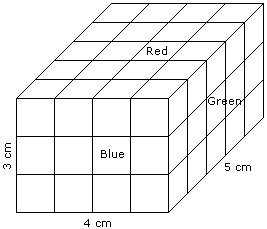Such cubes are related to the corners of the cuboid and in the cuboid there are 8 corners.

Hence, the required number of small cubes is 8.

Test: Cubes & Cuboids - Question 12

Directions to Solve

The following questions are based on the information given below:

1. A cuboid shaped wooden block has 4 cm length, 3 cm breadth and 5 cm height.
2. Two sides measuring 5 cm x 4 cm are coloured in red.
3. Two faces measuring 4 cm x 3 cm are coloured in blue.
4. Two faces measuring 5 cm x 3 cm are coloured in green.
5. Now the block is divided into small cubes of side 1 cm each.

Question -

How many small cubes will have only one face coloured ?

Detailed Solution for Test: Cubes & Cuboids - Question 122 from the front + 2 from the back + 3 from the left + 3 from the right + 6 from the top + 6 from the bottom = 22

Test: Cubes & Cuboids - Question 13

Directions to Solve

The following questions are based on the information given below:

1. A cuboid shaped wooden block has 4 cm length, 3 cm breadth and 5 cm height.
2. Two sides measuring 5 cm x 4 cm are coloured in red.
3. Two faces measuring 4 cm x 3 cm are coloured in blue.
4. Two faces measuring 5 cm x 3 cm are coloured in green.
5. Now the block is divided into small cubes of side 1 cm each.

Question -

How many small cubes will have no faces coloured ?

Detailed Solution for Test: Cubes & Cuboids - Question 13Required number of small cubes = (5 - 2) x (4 - 2) x (3 - 2)

= 3 x 2 x 1

= 6

Test: Cubes & Cuboids - Question 14

Directions to Solve

The following questions are based on the information given below:

1. A cuboid shaped wooden block has 4 cm length, 3 cm breadth and 5 cm height.
2. Two sides measuring 5 cm x 4 cm are coloured in red.
3. Two faces measuring 4 cm x 3 cm are coloured in blue.
4. Two faces measuring 5 cm x 3 cm are coloured in green.
5. Now the block is divided into small cubes of side 1 cm each.

Question -

How many small cubes will have two faces coloured with red and green colours ?

Detailed Solution for Test: Cubes & Cuboids - Question 14Required number of small cubes = 6 from the top and 6 from the bottom = 12

Test: Cubes & Cuboids - Question 15

Directions to Solve

The following questions are based on the information given below:

1. All the faces of cubes are painted with red colour.
2. The cubes is cut into 64 equal small cubes.

Question -

How many small cubes have only one face coloured ?

Detailed Solution for Test: Cubes & Cuboids - Question 15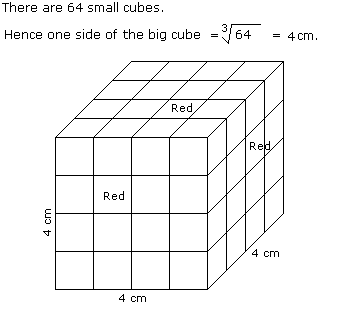Number of small cubes having only one face coloured = (x - 2)2 x No. of faces

= (4 - 2)2 x 6

= 24

Test: Cubes & Cuboids - Question 16

Directions to Solve

The following questions are based on the information given below:

1. All the faces of cubes are painted with red colour.
2. The cubes is cut into 64 equal small cubes.

Question -

How many small cubes have no faces coloured ?

Detailed Solution for Test: Cubes & Cuboids - Question 16Number of small cubes having only one faces coloured = (x - 2)3

Here, x = side of big cube / side of small cube

x = 4 /1

x = 4

Required number = (4 -2)3

= 8

Test: Cubes & Cuboids - Question 17

Directions to Solve

The following questions are based on the information given below:

1. All the faces of cubes are painted with red colour.
2. The cubes is cut into 64 equal small cubes.

Question -

How many small cubes are there whose three faces are coloured ?

Detailed Solution for Test: Cubes & Cuboids - Question 17Number of small cubes having three faces coloured = No. of corners = 8

Test: Cubes & Cuboids - Question 18

Directions to Solve

The following questions are based on the information given below:

1. All the faces of cubes are painted with red colour.
2. The cubes is cut into 64 equal small cubes.

Question -

How many small cubes are there whose two adjacent faces are coloured red ?

Detailed Solution for Test: Cubes & Cuboids - Question 18Number of small cubes having two adjacent faces coloured red = (x - 2) x No. of edges

= (4 - 2) x 12

= 24

Test: Cubes & Cuboids - Question 19

Directions to Solve

The following questions are based on the information given below:

All the opposite faces of a big cube are coloured with red, black and green colours. After that is cut into 64 small equal cubes.

Question -

How many small cubes are there whose no faces are coloured ?

Detailed Solution for Test: Cubes & Cuboids - Question 19Number of small cubes having no face coloured = (x - 2)3

= (4 - 2)3

= 8

Test: Cubes & Cuboids - Question 20

Directions to Solve

The following questions are based on the information given below:

All the opposite faces of a big cube are coloured with red, black and green colours. After that is cut into 64 small equal cubes.

Question -

How many small cubes are there whose 3 faces are coloured ?

Detailed Solution for Test: Cubes & Cuboids - Question 20Number of small cubes having three faces coloured = 1 at each corner

= 1 x 8

= 8

>

Test: Cubes & Cuboids - Question 21

Directions to Solve

The following questions are based on the information given below:

All the opposite faces of a big cube are coloured with red, black and green colours. After that is cut into 64 small equal cubes.

Question -

How many small cubes are there whose only one face is coloured ?

Detailed Solution for Test: Cubes & Cuboids - Question 21Number of small cubes having only one face coloured = 4 from each face

= 4 x 6

= 24

Test: Cubes & Cuboids - Question 22

Directions to Solve

The following questions are based on the information given below:

All the opposite faces of a big cube are coloured with red, black and green colours. After that is cut into 64 small equal cubes.

Question -

How many small cubes are there whose at the most two faces are coloured ?

Detailed Solution for Test: Cubes & Cuboids - Question 22Number of small cubes having two faces coloured = 8 + 8 + 4 + 4 = 24

and Number of small cubes having only one face coloured = 4 x 6 = 24

and Number of small cubes having no face coloured = 4 + 4 = 8

Therefore, total number of small cubes whose at the most two faces are coloured = 24 + 24 + 8 = 56.

Test: Cubes & Cuboids - Question 23

Directions to Solve

All the faces of a cube are painted with blue colour. Then it is cut into 125 small equal cubes.

Question -

How many small cubes will be formed having only one face coloured ?

Detailed Solution for Test: Cubes & Cuboids - Question 23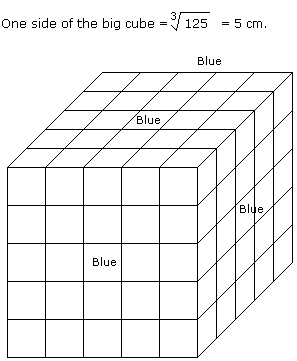No. of small cubes having only one face coloured = (5 - 2)2 x 6

= 9 x 6

= 54

Test: Cubes & Cuboids - Question 24

Directions to Solve

All the faces of a cube are painted with blue colour. Then it is cut into 125 small equal cubes.

Question -

How many small cubes will be formed having no face coloured ?

Detailed Solution for Test: Cubes & Cuboids - Question 24No. of small cubes having no face coloured = (x - 2)3

= (5 - 2)3

= 27

Test: Cubes & Cuboids - Question 25

Directions to Solve

All the six faces of a cube of a cube are coloured with six different colours - black, brown, green, red, white and blue.

1. Red face is opposite to the black face.
2. Green face is between red and black faces.
3. Blue face is adjacent to white face.
4. Brown face is adjacent to blue face.
5. Red face is in the bottom.

Question -

The upper face is _________

Detailed Solution for Test: Cubes & Cuboids - Question 25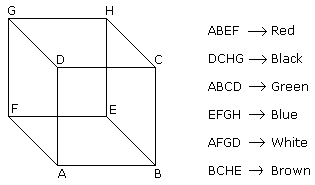Test: Cubes & Cuboids - Question 26

Directions to Solve

All the six faces of a cube of a cube are coloured with six different colours - black, brown, green, red, white and blue.

1. Red face is opposite to the black face.
2. Green face is between red and black faces.
3. Blue face is adjacent to white face.
4. Brown face is adjacent to blue face.
5. Red face is in the bottom.

Question -

Which of the following is adjacent to green ?

Detailed Solution for Test: Cubes & Cuboids - Question 26Test: Cubes & Cuboids - Question 27

Directions to Solve

All the six faces of a cube of a cube are coloured with six different colours - black, brown, green, red, white and blue.

1. Red face is opposite to the black face.
2. Green face is between red and black faces.
3. Blue face is adjacent to white face.
4. Brown face is adjacent to blue face.
5. Red face is in the bottom.

Question -

Which face is opposite to green ?

Detailed Solution for Test: Cubes & Cuboids - Question 27Test: Cubes & Cuboids - Question 28

Directions to Solve

There are 128 cubes with me which are coloured according to two schemes viz.

1. 64 cubes each having two red adjacent faces and one yellow and other blue on their opposite faces while green on the rest.
2. 64 cubes each having two adjacent blue faces and one red and other green on their opposite faces, while red on the rest. They are then mixed up.

Question -

How many cubes have at least two coloured red faces each ?

Detailed Solution for Test: Cubes & Cuboids - Question 28

64 and 64 cubes of both types of cubes are such who have at least two coloured faces red each.

Therefore, total number of the required cubes is 128.

Test: Cubes & Cuboids - Question 29

Directions to Solve

There are 128 cubes with me which are coloured according to two schemes viz.

1. 64 cubes each having two red adjacent faces and one yellow and other blue on their opposite faces while green on the rest.
2. 64 cubes each having two adjacent blue faces and one red and other green on their opposite faces, while red on the rest. They are then mixed up.

Question -

What is the total number of red faces ?

Detailed Solution for Test: Cubes & Cuboids - Question 29

No. of red faces among first 64 cubes = 128

No. of red faces among second 64 cubes = 192

Therefore, total number of red faces = 128 + 192 = 320

Test: Cubes & Cuboids - Question 30

Directions to Solve

There are 128 cubes with me which are coloured according to two schemes viz.

1. 64 cubes each having two red adjacent faces and one yellow and other blue on their opposite faces while green on the rest.
2. 64 cubes each having two adjacent blue faces and one red and other green on their opposite faces, while red on the rest. They are then mixed up.

Question -

Which two colours have the same number of faces ?

Detailed Solution for Test: Cubes & Cuboids - Question 30

First 64 cubes are such each of whose two faces are green and second 64 cubes are such each of whose two faces are blue.

Therefore, green and blue colours have the same number of faces.

Test: Cubes & Cuboids - Question 31

Directions to Solve

There are 128 cubes with me which are coloured according to two schemes viz.

1. 64 cubes each having two red adjacent faces and one yellow and other blue on their opposite faces while green on the rest.
2. 64 cubes each having two adjacent blue faces and one red and other green on their opposite faces, while red on the rest. They are then mixed up.

Question -

How many cubes have two adjacent blue faces each ?

Detailed Solution for Test: Cubes & Cuboids - Question 31

Second 64 cubes are such each of whose two faces are blue.

## Logical Reasoning (LR) and Data Interpretation (DI)

96 videos|90 docs|119 tests
 Use Code STAYHOME200 and get INR 200 additional OFF Use Coupon Code
Information about Test: Cubes & Cuboids Page
In this test you can find the Exam questions for Test: Cubes & Cuboids solved & explained in the simplest way possible. Besides giving Questions and answers for Test: Cubes & Cuboids, EduRev gives you an ample number of Online tests for practice

## Logical Reasoning (LR) and Data Interpretation (DI)

96 videos|90 docs|119 tests

### How to Prepare for CAT

Read our guide to prepare for CAT which is created by Toppers & the best Teachers